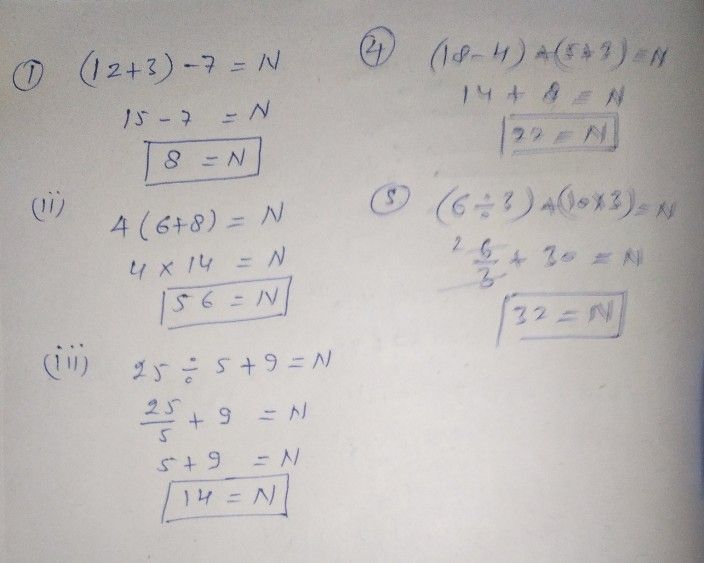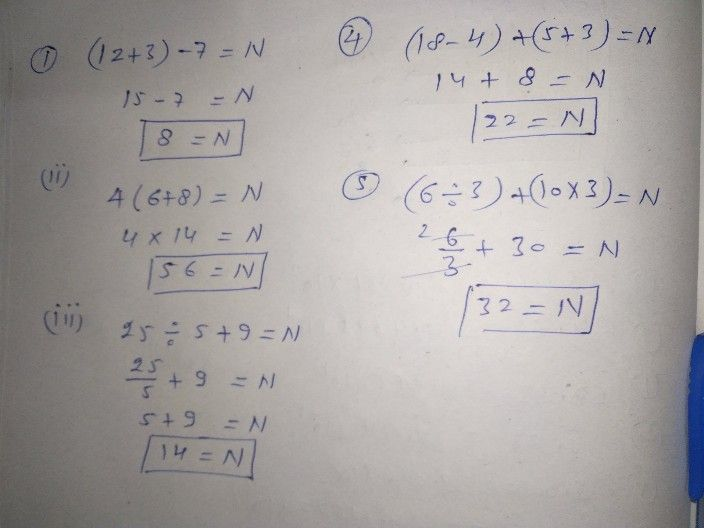Symbol
ProblemLearning Task $2°$ Perform the indicated operations. Write your answer in your notebook. $1.\right)$ $\left(12+3\right)$ $-7=N$ $4.\right)\left(18-4\right)$ $+\left(5+3\right)=N$ $2.\right)$ $416+8\right)=N$ $5.\right)$ $\left(6\div 3\right)+\left(10\times 3\right)=N$ $3.\right)$ $25\div 5+9=N$